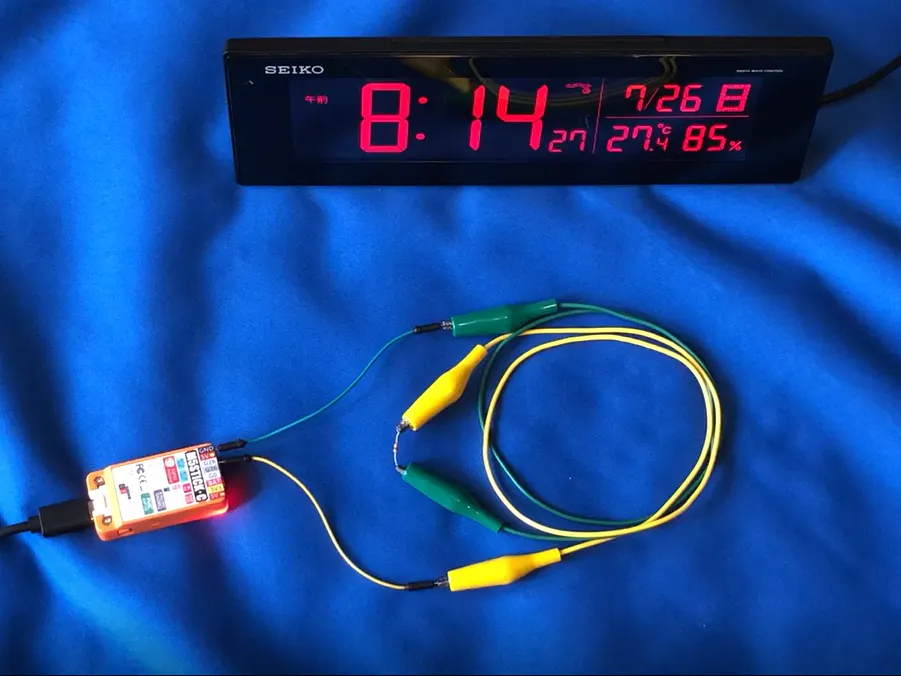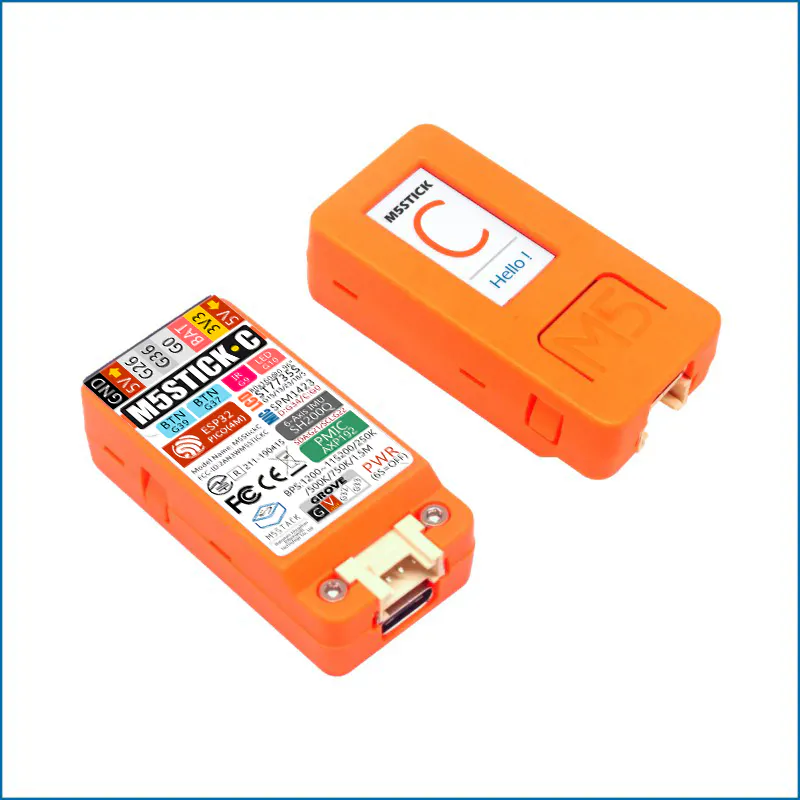# Generate Standard Radio Wave JJY with Ticker of M5StickC

Transit NTP acquisition time short-distance transmission within weak distance.

IntermediateFull instructions provided2 hours8## Things used in this project

### Hardware componentsM5Stack M5StickC ESP32-PICO Mini IoT Development Board
×1

## Code

### JJY Simulator for M5StickC

C/C++
```// copyright 2020 BotanicFields, Inc.
// BF-018
// JJY Simulator for M5StickC
//
#include <M5StickC.h>
#include <Ticker.h>
#include <WiFi.h>
#include <WiFiManager.h> // https://github.com/tzapu/WiFiManager

// for NTP and time
const long  GMTOFFSET = 3600 * 9;  // JST-9
const int   DAYLIGHT  = 3600 * 0;  // No daylight time
const char* NTPSERVER = "pool.ntp.org";
struct tm timeinfo;
struct timeval tv_now;

// for LEDC(PWM)
const uint8_t  LEDC_PIN      = 26;
const uint8_t  LEDC_CH       = 0;
const double   LEDC_FREQ     = 4e4;  // 40kHz
const uint8_t  LEDC_RESO     = 10;   // 2^10 = 1024
const uint32_t LEDC_DUTY_ON  = 512;  // 50%
const uint32_t LEDC_DUTY_OFF = 0;    // 0

// for monitoring
const int LED  = 10;      // LED to monitor
bool led_enable = true;   //

// for TCO(Time Code Output)
const int TK_MS  = 100;   // Ticker period (ms)
const int MARKER = 0xff;  // MARKER code which TCO_val() returns
long last_us;             // for statistics of Ticker
Ticker tk;

void TCO_gen() {
if(!getLocalTime(&timeinfo)) {
Serial.println("[TCO]Failed to obtain time");
return;
}

gettimeofday(&tv_now, NULL);
long tv_100ms = tv_now.tv_usec / 100000L;
switch(tv_100ms) {
case  0: TCO_000ms(); break;
case  2: TCO_200ms(); break;
case  5: TCO_500ms(); break;
case  8: TCO_800ms(); break;
default: break;
}

// statistics of tv_usec
long now_us = tv_now.tv_usec;
if(now_us < 100000L) now_us += 1000000L;
long diff_us = now_us - last_us - 100000L;
last_us = tv_now.tv_usec;

static long diff_num = 0L;
static long diff_max = 0L;
static long diff_min = 0L;
static double diff_sum = 0.0;
static double diff_sum_sq = 0.0;
diff_num++;
if(diff_max < diff_us) diff_max = diff_us;
if(diff_min > diff_us) diff_min = diff_us;
diff_sum += (double)diff_us;
diff_sum_sq += (double)diff_us * (double)diff_us;

static long dist = {0L, 0L, 0L, 0L, 0L, 0L, 0L, 0L, 0L};
if(     diff_us < -50000) dist++;  // < -50000us(< -50ms)
else if(diff_us <  -5000) dist++;  //  < -5000us(<  -5ms)
else if(diff_us <   -500) dist++;  //   < -500us
else if(diff_us <    -50) dist++;  //    < -50us
else if(diff_us <     50) dist++;  //   -50~50us
else if(diff_us <    500) dist++;  //    >  50us
else if(diff_us <   5000) dist++;  //   >  500us
else if(diff_us <  50000) dist++;  //  >  5000us(>   5ms)
else                      dist++;  // >  50000us(>  50ms)

if((timeinfo.tm_sec == 0) && (tv_now.tv_usec < 100000)) {
for(int i = 0; i < 9; i++) {
Serial.printf("%d ", dist[i]);
}
Serial.println();

double diff_ave = diff_sum / (double)diff_num;
double diff_var = (diff_sum_sq - diff_sum * diff_sum / (double)diff_num) / (double)diff_num;
double diff_sdv = sqrt(diff_var);
Serial.printf("n= %d, ave= %.4f  sdv= %.4f  min= %d  max= %d\n", diff_num, diff_ave, diff_sdv, diff_min, diff_max);
}
}

// TCO task at every 0ms
void TCO_000ms() {
TC_on();

if(timeinfo.tm_sec == 0) {
Serial.println();
Serial.println(&timeinfo, "%A %B %d %Y %H:%M:%S  ");
}
}

// TCO task at every 200ms
void TCO_200ms() {
if(TC_val() == MARKER) {
TC_off();

Serial.print(" ");
Serial.print(timeinfo.tm_sec);
Serial.print(" ");
}
}

// TCO task at every 500ms
void TCO_500ms() {
if(TC_val() != 0) {
TC_off();

if(TC_val() != MARKER) {
Serial.print("1");
}
}
}

// TCO task at every 800ms
void TCO_800ms() {
TC_off();

if(TC_val() == 0) {
Serial.print("0");
}
}

void TC_on() {
ledcWrite(LEDC_CH, LEDC_DUTY_ON);
if(led_enable) digitalWrite(LED, LOW);
}

void TC_off() {
ledcWrite(LEDC_CH, LEDC_DUTY_OFF);
digitalWrite(LED, HIGH);
}

// TCO value
//   MARKER, 1:not zero, 0:zero
int TC_val() {
int h = int3bcd(timeinfo.tm_hour);
int parity_h = parity8(h);
int m = int3bcd(timeinfo.tm_min);
int parity_m = parity8(m);
int year = timeinfo.tm_year + 1900;
int y = int3bcd(year);

const int MONTH = { 31, 28, 31, 30, 31, 30, 31, 31, 30, 31, 30, 31 };
int days = timeinfo.tm_mday;
for(int i = 0; i < timeinfo.tm_mon; i++) {  // timeinfo.tm_mon starts from 0
days += MONTH[i];
}
if((timeinfo.tm_mon > 1) && ((year % 4 == 0) && (year % 100 != 0)) || (year % 400 == 0)) {
days++;
}
int d = int3bcd(days);

int w = timeinfo.tm_wday;

int tc;
switch(timeinfo.tm_sec) {
case  0: tc = MARKER;     break;
case  1: tc = m & 0x40;   break;
case  2: tc = m & 0x20;   break;
case  3: tc = m & 0x10;   break;
case  4: tc = 0;          break;
case  5: tc = m & 0x08;   break;
case  6: tc = m & 0x04;   break;
case  7: tc = m & 0x02;   break;
case  8: tc = m & 0x01;   break;
case  9: tc = MARKER;     break;

case 10: tc = 0;          break;
case 11: tc = 0;          break;
case 12: tc = h & 0x20;   break;
case 13: tc = h & 0x10;   break;
case 14: tc = 0;          break;
case 15: tc = h & 0x08;   break;
case 16: tc = h & 0x04;   break;
case 17: tc = h & 0x02;   break;
case 18: tc = h & 0x01;   break;
case 19: tc = MARKER;     break;

case 20: tc = 0;          break;
case 21: tc = 0;          break;
case 22: tc = d & 0x200;  break;
case 23: tc = d & 0x100;  break;
case 24: tc = 0;          break;
case 25: tc = d & 0x080;  break;
case 26: tc = d & 0x040;  break;
case 27: tc = d & 0x020;  break;
case 28: tc = d & 0x010;  break;
case 29: tc = MARKER;     break;

case 30: tc = d & 0x008;  break;
case 31: tc = d & 0x004;  break;
case 32: tc = d & 0x002;  break;
case 33: tc = d & 0x001;  break;
case 34: tc = 0;          break;
case 35: tc = 0;          break;
case 36: tc = parity_h;   break;
case 37: tc = parity_m;   break;
case 38: tc = 0;          break;
case 39: tc = MARKER;     break;

case 40: tc = 0;          break;
case 41: tc = y & 0x80;   break;
case 42: tc = y & 0x40;   break;
case 43: tc = y & 0x20;   break;
case 44: tc = y & 0x10;   break;
case 45: tc = y & 0x08;   break;
case 46: tc = y & 0x04;   break;
case 47: tc = y & 0x02;   break;
case 48: tc = y & 0x01;   break;
case 49: tc = MARKER;     break;

case 50: tc = w & 0x04;   break;
case 51: tc = w & 0x02;   break;
case 52: tc = w & 0x01;   break;
case 53: tc = 0;          break;
case 54: tc = 0;          break;
case 55: tc = 0;          break;
case 56: tc = 0;          break;
case 57: tc = 0;          break;
case 58: tc = 0;          break;
case 59: tc = MARKER;     break;
default: tc = 0;          break;
}
return tc;
}

int int3bcd(int a) {
return (a % 10) + (a / 10 % 10 * 16) + (a / 100 % 10 * 256);
}

int parity8(int a) {
int pa = a;
for(int i = 1; i < 8; i++) {
pa += a >> i;
}
return pa % 2;
}

// LCD display
const int UPDATE_INTERVAL =  100;                    // delay in ms
const int SHOW_DULATION   = 5000 / UPDATE_INTERVAL;  // dulation in ms / INTERVAL
int showRemain = 0;

void showSetup() {
M5.Lcd.setRotation(1);
M5.Lcd.fillScreen(BLACK);
M5.Axp.ScreenBreath(7);
}

void showStatus(bool show_yes) {
if(show_yes) {
M5.Axp.ScreenBreath(10);
M5.Lcd.setTextSize(1);
M5.Lcd.setCursor(0, 0, 2);
M5.Lcd.setTextColor(WHITE, DARKGREEN);
M5.Lcd.println("    BotanicFields, Inc.   ");

M5.Lcd.setTextColor(WHITE, BLACK);
M5.Lcd.println(WiFi.SSID());
M5.Lcd.println(WiFi.localIP().toString());

if(!getLocalTime(&timeinfo)) {
M5.Lcd.println("Failed to obtain time");
}
else {
M5.Lcd.println(&timeinfo, "%B %d %Y");
M5.Lcd.println(&timeinfo, "%A %H:%M:%S");
}
M5.Lcd.setCursor(120, 32, 2);
if(led_enable) {
M5.Lcd.println("L-on ");
}
else {
M5.Lcd.println("L-off");
}
}
else {
M5.Lcd.fillScreen(BLACK);
M5.Axp.ScreenBreath(7);
}
}

// for RTC .. not used actually
void RTCset() {
RTC_TimeTypeDef TimeStruct;
TimeStruct.Hours   = timeinfo.tm_hour;
TimeStruct.Minutes = timeinfo.tm_min;
TimeStruct.Seconds = timeinfo.tm_sec;
M5.Rtc.SetTime(&TimeStruct);
RTC_DateTypeDef DateStruct;
DateStruct.WeekDay = timeinfo.tm_wday;
DateStruct.Month   = timeinfo.tm_mon + 1;
DateStruct.Date    = timeinfo.tm_mday;
DateStruct.Year    = timeinfo.tm_year + 1900;
M5.Rtc.SetData(&DateStruct);
}

void setup() {
M5.begin();

// setup Wifi
WiFi.mode(WIFI_STA);
WiFiManager wm;
bool res = wm.autoConnect();  // auto generated AP name from chipid
// bool res = wm.autoConnect("AutoConnectAP"); // anonymous ap

if(!res)
Serial.println("Failed to connect");
else
Serial.println("connected...yeey :)");

// start NTP
configTime(GMTOFFSET, DAYLIGHT, NTPSERVER);
while(!getLocalTime(&timeinfo)) {
Serial.println("Failed to obtain time");
delay(100);
}
Serial.println(&timeinfo, "%A %B %d %Y %H:%M:%S");
RTCset();  // The RTC is not used anyway.

// start LEDC
ledcSetup(LEDC_CH, LEDC_FREQ, LEDC_RESO);
ledcAttachPin(LEDC_PIN, LEDC_CH);

// for monitoring
pinMode(LED, OUTPUT);

// wait until middle of 100ms timing. ex. 50ms, 150ms, 250ms,..
gettimeofday(&tv_now, NULL);
delayMicroseconds(150000L - tv_now.tv_usec % 100000L);

// for statistics of first sample
gettimeofday(&tv_now, NULL);
last_us = tv_now.tv_usec;

// start TCO
tk.attach_ms(TK_MS, TCO_gen);

// for main loop
showSetup();
}

void loop() {
M5.update();

// button-A: LCD-on for a while
if(M5.BtnA.wasReleased()) {
showStatus(true);
showRemain = SHOW_DULATION;
}
else if(showRemain != 0) {
showStatus(true);
showRemain--;
}
else {
showStatus(false);
}

// button-B: LED monitor on/off
if(M5.BtnB.wasReleased()) {
led_enable = !led_enable;
}

// loop delay
delay(UPDATE_INTERVAL);
}

/*
SPDX short identifier: MIT
Permission is hereby granted, free of charge, to any person obtaining a copy
of this software and associated documentation files (the "Software"), to deal
in the Software without restriction, including without limitation the rights
to use, copy, modify, merge, publish, distribute, sublicense, and/or sell
copies of the Software, and to permit persons to whom the Software is
furnished to do so, subject to the following conditions:
The above copyright notice and this permission notice shall be included in all
copies or substantial portions of the Software.
THE SOFTWARE IS PROVIDED "AS IS", WITHOUT WARRANTY OF ANY KIND, EXPRESS OR
IMPLIED, INCLUDING BUT NOT LIMITED TO THE WARRANTIES OF MERCHANTABILITY,
FITNESS FOR A PARTICULAR PURPOSE AND NONINFRINGEMENT. IN NO EVENT SHALL THE
AUTHORS OR COPYRIGHT HOLDERS BE LIABLE FOR ANY CLAIM, DAMAGES OR OTHER
LIABILITY, WHETHER IN AN ACTION OF CONTRACT, TORT OR OTHERWISE, ARISING FROM,
OUT OF OR IN CONNECTION WITH THE SOFTWARE OR THE USE OR OTHER DEALINGS IN THE
SOFTWARE.
*/
```

BotanicFields